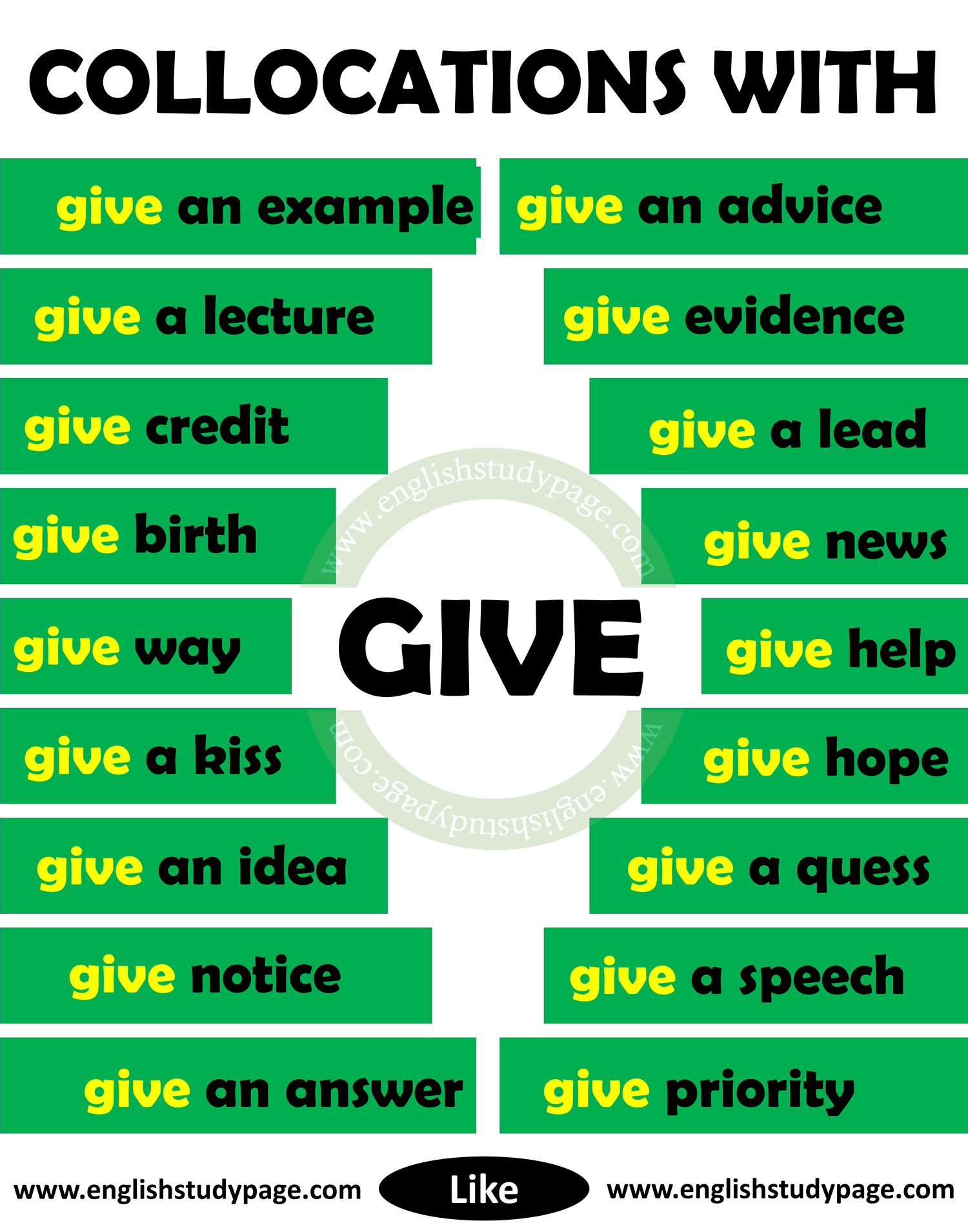# Collocations With GIVE in English

Collocations list in English. Please follow the list;Collocation” means a group of words which usually go together. To reach detailed collocation list with give in english, follow the list;

 Collocations With GIVE give a call give a chance give a damn give a hand give a choice give a headache give a hug give a kiss give a ring give a description give a lift give a lecture give a speech give a performance give a ride give an advice give an answer give an example give an idea give a buzz give a quess give a trip give an opinion give birth give credit give evidence give notice give permission give priority give rise to give information give a lead give lessons give the impression give thought (to) give hope give way give somebody a call give somebody a chance give somebody a lift give somebody a go give news give an explanation give help